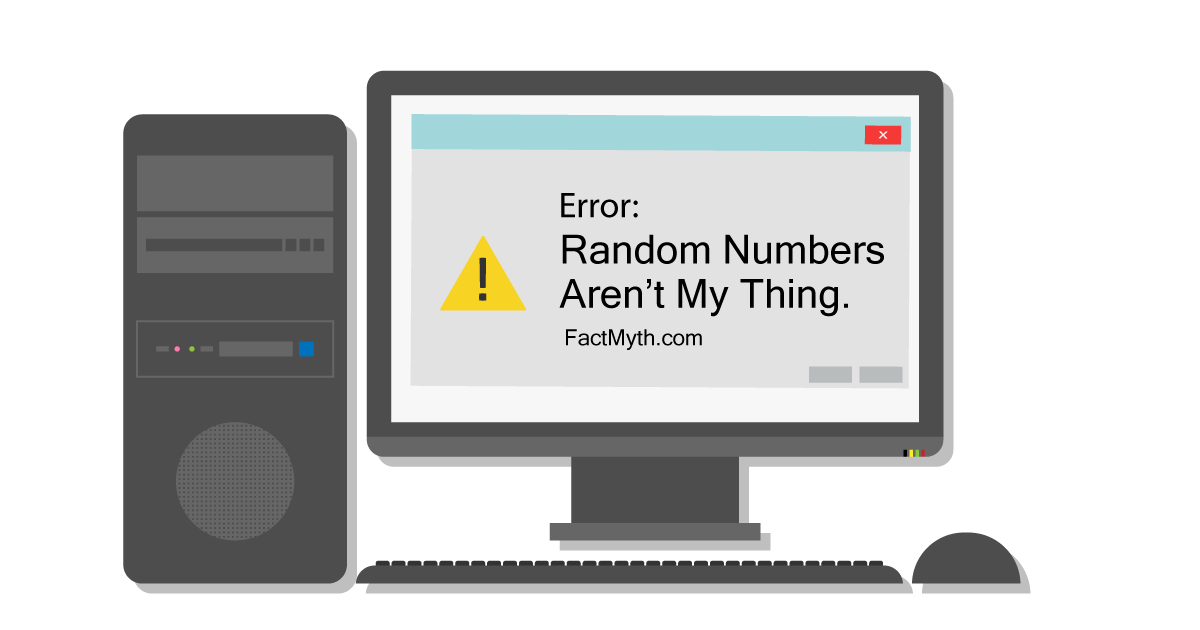# There is a Difference Between Chaos and Randomness factThere is a difference between chaos and randomness, and it can be proved with mathematics. Chaos is deterministic, while true randomness is non-deterministic.

First, let me spare you the math and explain things in simple terms (see the citations below for the math).

Chaos has an order and pattern and is dependent on initial conditions. No matter how irregular and disorderly an outcome, there is an underlying order. The results can be recreated reliably given a certain input.

For example, if a pool cue is used to hit pool balls, the results might be chaotic, but not random. Knowing the results one could recreate the event by hitting the pool balls in the exact same way with the exact same setup. This is how classic physics works.

Chaos Wikipedia Definition: Dynamical systems whose apparently random states of disorder and irregularities are actually governed by underlying patterns and deterministic laws that are highly sensitive to initial conditions.

True randomness has no order and does not follow any pattern. No matter the outcome, there is no order. The results cannot be recreated reliably given a certain input.

For example, if a random amount of pool balls appeared on a table in a random pattern using a magic pool ball generator, there would be no reliable way to recreate the sequence. This is how quantum physics works.

Randomness Wikipedia definition: Randomness is the apparent lack of pattern or predictability in events. A random sequence of events, symbols, or steps often has no order and does not follow an intelligible pattern or combination.

Modulation: Periodic vs Random vs Chaotic. An interesting way to wrap your head around the difference between chaotic and random by using periodic sequence generation as a control.

1. What is the Difference Between Random and Chaotic Sequences [PDF]. Math.Tamu.Edu.
2. What is the difference between chaos and limited random function? ResearchGate.Net.
3. Chaos theory. Wikipedia.org.
4. Randomness. Wikipedia.org.